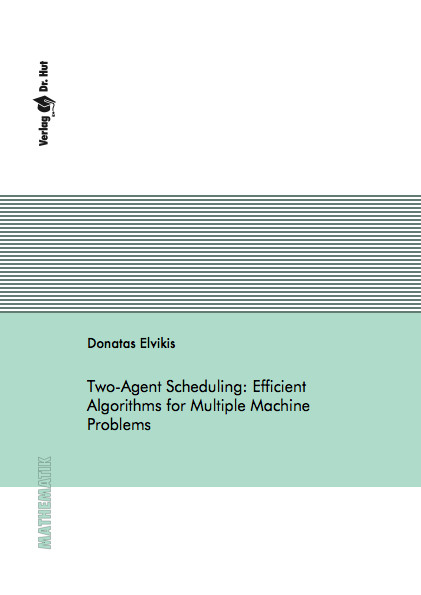Datenbestand vom 20. August 2019aktualisiert am 20. August 2019

# ISBN 9783843903622Euro 60,00 inkl. 7% MwSt

978-3-8439-0362-2, Reihe Mathematik

Donatas Elvikis
Two-Agent Scheduling: Efficient Algorithms for Multiple Machine Problems

140 Seiten, Dissertation Technische Universität Kaiserslautern (2011), Softcover, A5

## Zusammenfassung / Abstract

In this thesis we consider scheduling problems with two agents A and B where each agent has its own set of jobs to be scheduled on common resources. Agents are assumed to be independent from each other and have n_A and n_B jobs, respectively. We focus on developing polynomial time algorithms which can efficiently enumerate a complete minimal Pareto set P. In particular, instead of naive application of, e.g., epsilon-constraint method we develop sophisticated techniques which allow us for some specific problems to move from one nondominated point to a succeeding one.

Concerning machine environment, we focus on two types of problems, namely uniform parallel machines and flowshop scheduling. In the first case we limit ourselves to the problems characterized by jobs with equal processing times independent of agent the job belongs to. Based on derived properties we reduce considered problems to finding a proper jobs assignment to the free timeslots. Moreover, we show how some problems can be efficiently enumerated without a need to start building every new solution from the very beginning for each nondominated point. We develop algorithms for problems with both criteria associated with general nondecreasing maximum functions as well as makespan and some rather general sum cost functions.

For the two machine flowshop problems we consider a case where all jobs belonging to the same agent have equal processing times on the second machine. Derived algorithms use a property that there is always an efficient schedule such that jobs of one agent are ordered with respect to the shortest processing times on the first machine if makespan or total flow time is minimized. If agent B minimizes the makespan we derive an equation which computes a minimal amount of idle time in schedule if some or all jobs of agent A complete before the makespan of agent B. Based on this we derive a greedy type algorithm to efficiently solve total flow time and makespan criteria problems. In case both agents minimize the makespan we show that for each agent we need to consider at most two subsets of jobs which always form a continuous block in an efficient schedule.